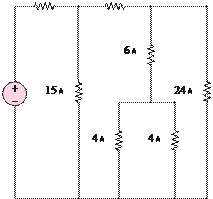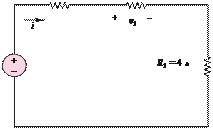### Create an Account

Home / Questions / In the circuit of Figure P246 the power absorbed by the 15Q resistor is 15 W Find R R...

# In the circuit of Figure P246 the power absorbed by the 15Q resistor is 15 W Find R R 4 Ù c Figure P242 2 Ù 6 Ù 25 V

In the circuit of Figure P2.46, the power absorbed by the 15-resistor is 15 W. Find R.

R                    4 Ù

(c)

Figure P2.42

2 Ù                               6 Ù25 V

6 V

Figure P2.43_

v2

+

Figure P2.46

Jun 19 2020 View more View LessSubscribe To Get Solution